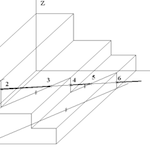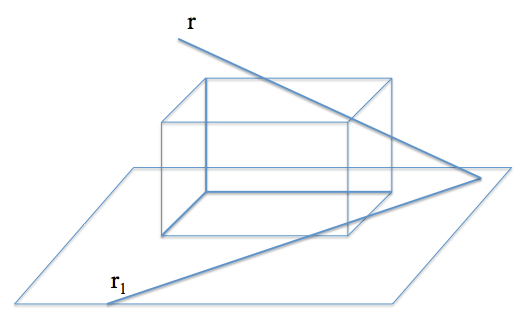# Intersections in outlook: straight and cuboidsOne of the classic problems of representation systems is to find the intersection of two elements, such as determining the intersection point between a line and a plane. Topological nature are problems in which the concepts of belonging prevail.

The problems are based on topological relationships are independent projection type in which they are.

Intersection problems are based on determining the geometric elements that belong to two elements simultaneously, considering that these are composed of infinite points.

We will study the example study the following problem:

Find all points of intersection of the line r with the given prism isometric, indicating the visibility of the line. To solve this problem previously remember within incidence problems, determining the point of intersection between a line and a plane.We have seen the general model for determining intersections systems representation. In this approach, have a auxiliary plane containing the given line and we will produce a straight intersection with the plane.In this kind of problems, when the intersection is a prism, we consistently repeat the problem of intersection between planes. For example we can resolve in the first instance intersection of a line r with a cuboid (a box with sides parallel and perpendicular). The line will be determined by direct projection (r) and the projection onto the plane of the base (r1)This will determine a plane containing the straight, in this case, is orthogonal to the base. This will produce a straight plane intersects with the body that contain the points of intersection.

• The determination of these lines intersect will be based on the conditions of parallelism between the different planes of the body, as a plane sectioned according to two parallel lines parallel planes.
• If the plane is perpendicular to the base, Others junction with the planes perpendicular to this will determine rectas Verticales.If the body is more complex than we resolve the intersections with some more work, although the overall pattern will be the same if the faces remain parallel to the projection.

For the resolution of this exercise, first determining section that causes the plane containing the straight line r and their projection on the horizontal plane XY (r1), given on prismatic. The entry and exit points (1, 2, 3, 4, 5 and 6) are obtained as points of intersection of the line with said section r.We will see later how to resolve cases in which it faces oblique.